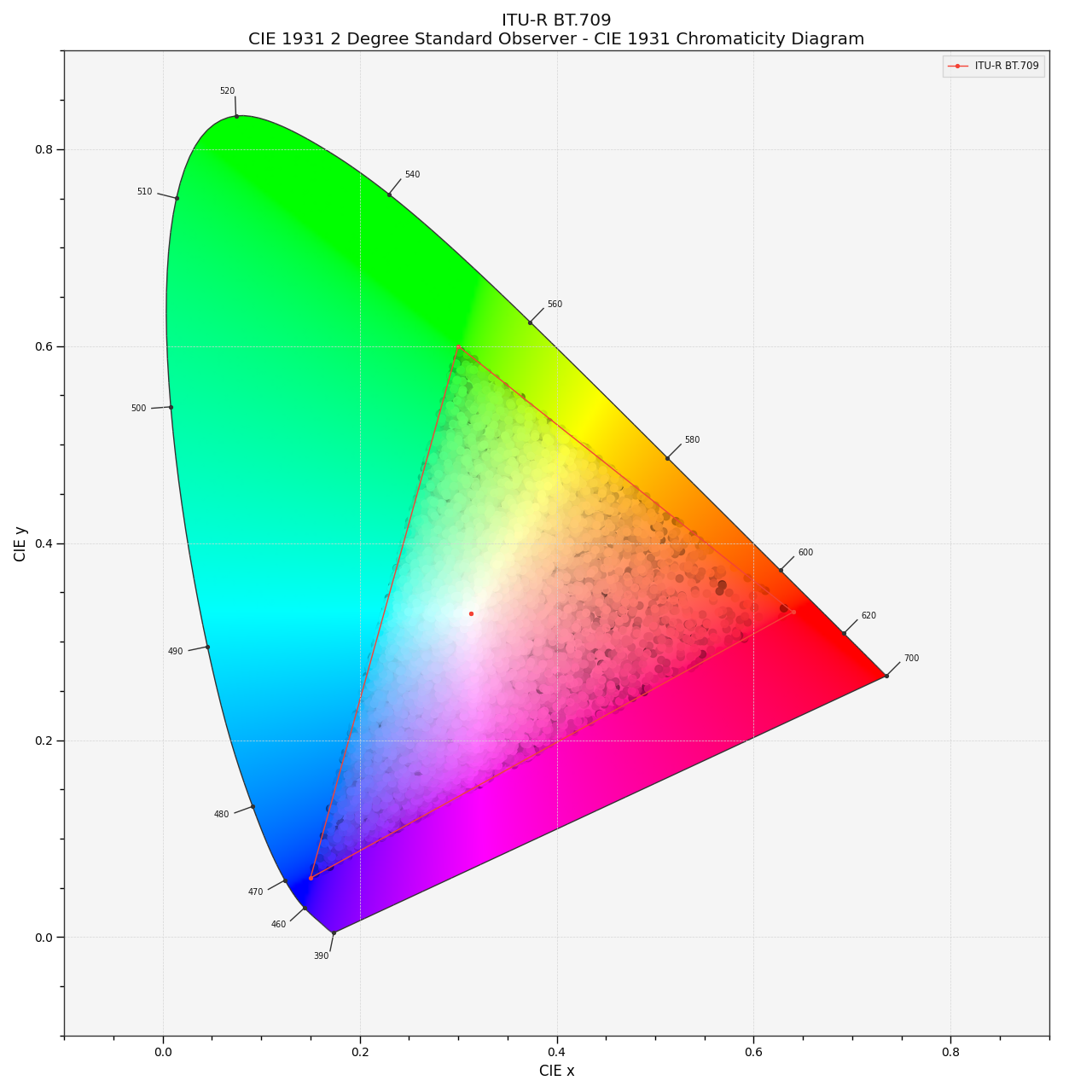# colour.plotting.models.plot_RGB_chromaticities_in_chromaticity_diagram¶

colour.plotting.models.plot_RGB_chromaticities_in_chromaticity_diagram(RGB, colourspace='sRGB', chromaticity_diagram_callable=<function plot_RGB_colourspaces_in_chromaticity_diagram>, method='CIE 1931', scatter_kwargs=None, **kwargs)[source]

Plots given RGB colourspace array in the Chromaticity Diagram according to given method.

Parameters
• RGB (array_like) – RGB colourspace array.

• colourspace (unicode or RGB_Colourspace, optional) – RGB colourspace of the RGB array. `colourspace` can be of any type or form supported by the `colour.plotting.filter_RGB_colourspaces()` definition.

• chromaticity_diagram_callable (callable, optional) – Callable responsible for drawing the Chromaticity Diagram.

• method (unicode, optional) – {‘CIE 1931’, ‘CIE 1960 UCS’, ‘CIE 1976 UCS’}, Chromaticity Diagram method.

• scatter_kwargs (dict, optional) –

Keyword arguments for the `plt.scatter()` definition. The following special keyword arguments can also be used:

• c : unicode or array_like, if `c` is set to RGB, the scatter will use the colours as given by the `RGB` argument.

• **kwargs (dict, optional) – {`colour.plotting.artist()`, `colour.plotting.diagrams.plot_chromaticity_diagram()`, `colour.plotting.diagrams.plot_RGB_colourspaces_in_chromaticity_diagram()`, `colour.plotting.render()`}, Please refer to the documentation of the previously listed definitions.

Returns

Current figure and axes.

Return type

tuple

Examples

```>>> RGB = np.random.random((128, 128, 3))
>>> plot_RGB_chromaticities_in_chromaticity_diagram(
...     RGB, 'ITU-R BT.709')
...
(<Figure size ... with 1 Axes>, <...AxesSubplot...>)
```